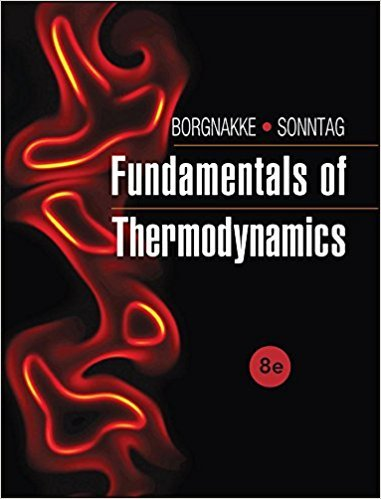×
Log in to StudySoup
Get Full Access to Fundamentals Of Thermodynamcs - 8 Edition - Chapter 5 - Problem 2cgp
Join StudySoup for FREE
Get Full Access to Fundamentals Of Thermodynamcs - 8 Edition - Chapter 5 - Problem 2cgp

Already have an account? Login here
×
Reset your password

# Compare two domestic heat pumps (A and B) running with theISBN: 9781118131992 58

## Solution for problem 2CGP Chapter 5

Fundamentals of Thermodynamcs | 8th Edition

• Textbook Solutions
• 2901 Step-by-step solutions solved by professors and subject experts
• Get 24/7 help from StudySoup virtual teaching assistantsFundamentals of Thermodynamcs | 8th Edition

4 5 1 278 Reviews
11
5
Problem 2CGP

Problem 2CGP

Compare two domestic heat pumps (A and B) running with the same work input. If A is better than B, which one provides more heat?

Step-by-Step Solution:

Solution 2CGP

Step 1 of 3

The objective here is to evaluate which heat pump produces more heat when two heat pumps are provided with the same heat and one heat pump is better performing than the other.

Step 2 of 3

Step 3 of 3

##### ISBN: 9781118131992

The full step-by-step solution to problem: 2CGP from chapter: 5 was answered by , our top Engineering and Tech solution expert on 08/03/17, 05:05AM. This full solution covers the following key subjects: heat, Input, Compare, domestic, better. This expansive textbook survival guide covers 7 chapters, and 1462 solutions. This textbook survival guide was created for the textbook: Fundamentals of Thermodynamcs , edition: 8. Since the solution to 2CGP from 5 chapter was answered, more than 570 students have viewed the full step-by-step answer. Fundamentals of Thermodynamcs was written by and is associated to the ISBN: 9781118131992. The answer to “Compare two domestic heat pumps (A and B) running with the same work input. If A is better than B, which one provides more heat?” is broken down into a number of easy to follow steps, and 25 words.

Unlock Textbook Solution

Enter your email below to unlock your verified solution to:

Compare two domestic heat pumps (A and B) running with the